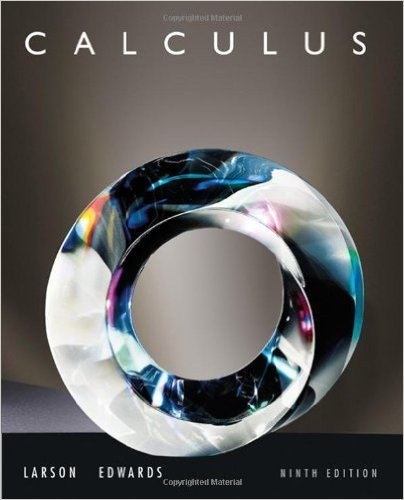×
×

# Solutions for Chapter 1.4: Continuity and One-Sided Limits## Full solutions for Calculus | 9th Edition

ISBN: 9780547167022Solutions for Chapter 1.4: Continuity and One-Sided Limits

Solutions for Chapter 1.4
4 5 0 409 Reviews
12
0
##### ISBN: 9780547167022

Chapter 1.4: Continuity and One-Sided Limits includes 122 full step-by-step solutions. This expansive textbook survival guide covers the following chapters and their solutions. This textbook survival guide was created for the textbook: Calculus , edition: 9. Since 122 problems in chapter 1.4: Continuity and One-Sided Limits have been answered, more than 63212 students have viewed full step-by-step solutions from this chapter. Calculus was written by and is associated to the ISBN: 9780547167022.

Key Calculus Terms and definitions covered in this textbook
• Complex plane

A coordinate plane used to represent the complex numbers. The x-axis of the complex plane is called the real axis and the y-axis is the imaginary axis

• Directed line segment

See Arrow.

• Directrix of a parabola, ellipse, or hyperbola

A line used to determine the conic

• Double-angle identity

An identity involving a trigonometric function of 2u

• Geometric sequence

A sequence {an}in which an = an-1.r for every positive integer n ? 2. The nonzero number r is called the common ratio.

• Higher-degree polynomial function

A polynomial function whose degree is ? 3

See Polynomial function in x.

• Length of an arrow

See Magnitude of an arrow.

• Limaçon

A graph of a polar equation r = a b sin u or r = a b cos u with a > 0 b > 0

• Logistic regression

A procedure for fitting a logistic curve to a set of data

• LRAM

A Riemann sum approximation of the area under a curve ƒ(x) from x = a to x = b using x1 as the left-hand endpoint of each subinterval

• Midpoint (in a coordinate plane)

For the line segment with endpoints (a,b) and (c,d), (aa + c2 ,b + d2)

• Natural logarithmic regression

A procedure for fitting a logarithmic curve to a set of data.

• Paraboloid of revolution

A surface generated by rotating a parabola about its line of symmetry.

• Random numbers

Numbers that can be used by researchers to simulate randomness in scientific studies (they are usually obtained from lengthy tables of decimal digits that have been generated by verifiably random natural phenomena).

• Scalar

A real number.

• Second

Angle measure equal to 1/60 of a minute.

• Solve algebraically

Use an algebraic method, including paper and pencil manipulation and obvious mental work, with no calculator or grapher use. When appropriate, the final exact solution may be approximated by a calculator

• Solve by elimination or substitution

Methods for solving systems of linear equations.

• Third quartile

See Quartile.

×